# An Anecdote of Averages!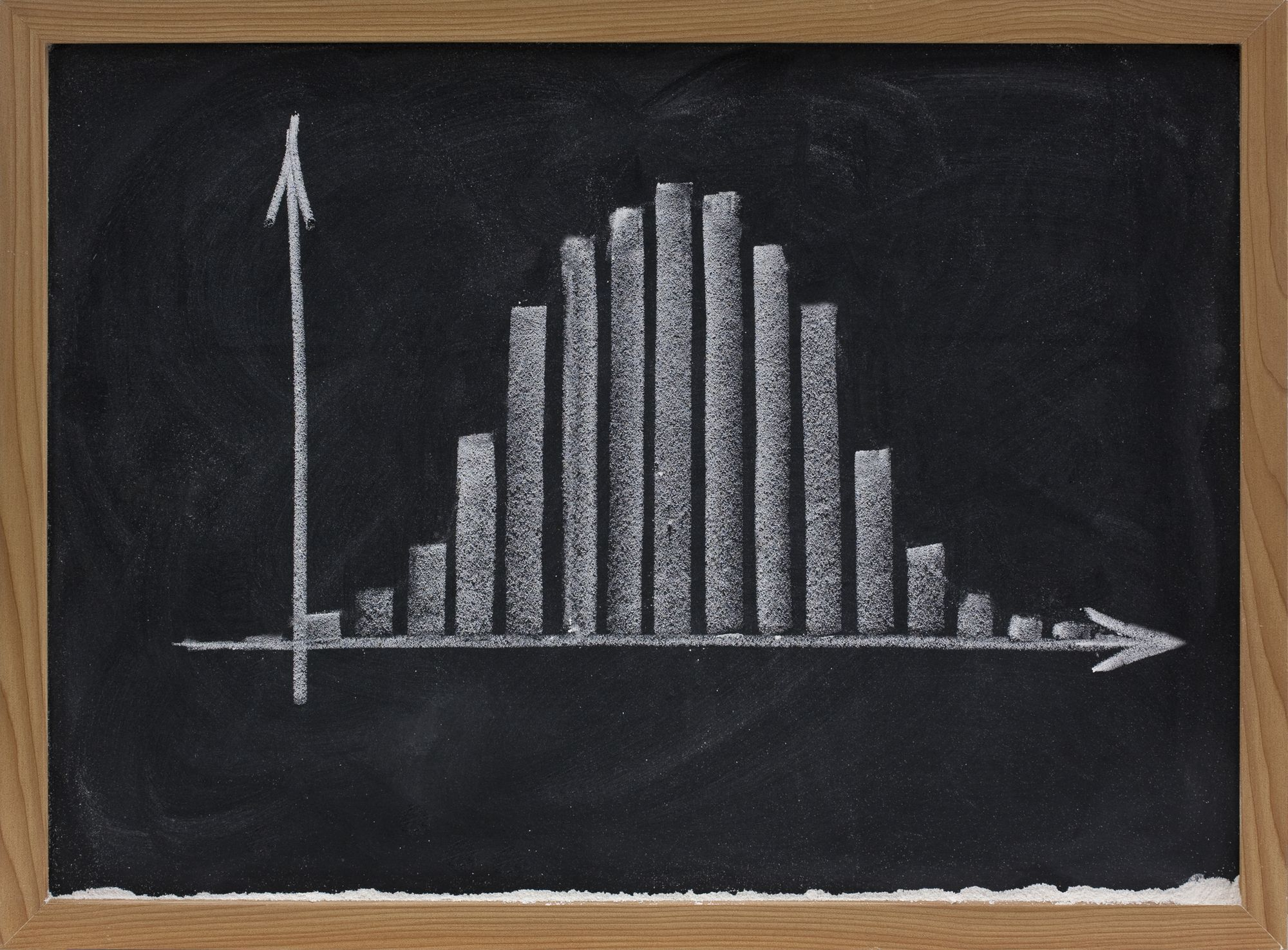Get to know the 3 M’s – Mean, Median, Mode of statistics a little deeper, they trick you more so often.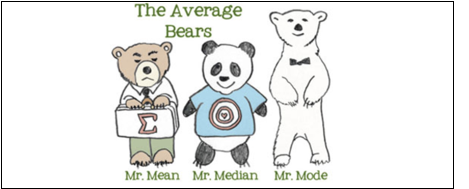We encounter sentences like “The average salary of a teacher in the..”, almost on a daily basis, in newspapers, articles, reports etc. The number that is being reported as the so called average is not of much value to us and we cannot interpret much from it, as to what it really means, unless we figure out which one of the common kinds of average is being used in the context.

Mean, Median and Mode are the three common kinds of averages, each of them have a different mathematical formula and a different interpretation. These three are also called as the measures of central tendency. So let us have a quick walkthrough.

Mean: We are all familiar with all the three averages, but somehow, many of us think of only the “mean”, when we hear the word “average”.  That’s how popular this guy is.

Mean has a straightforward formula and that is,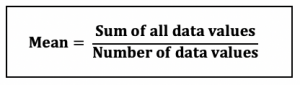Median: The median is the middle value of a data set. The catch here is that the dataset (list of values) has to be ordered first, from lowest to highest value.

The number of values, let us denote it as n, in the dataset could be even or odd.

If n = odd, it’s pretty straightforward, as there is only one middle value.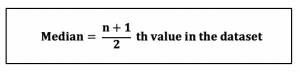If n = even, the median will be the mean of the middle two values in the dataset.

Mode: The  mode is the most common value, the one that occurs most frequently in the dataset.

Let us get a better idea using a simple example.

Dataset = {100,200,300,300,400,500}

Here, n = 6

Mean = ( 100+200+300+300+400+500)/6 = 300

Median = (300+300)/2 = 300 (the mean of the middle two numbers, since n is even)

Mode = 300 (it appears twice, while the other numbers are present only once)

Things are always not as simple as we want them to be.
Let us now get to know the effect of outliers on the measures of average.

The same example dataset with only one number changed: {100,200,300,300,400,1000}

If you take a look at the dataset, compared to the other values, 1000 is a bit far and bigger. So it is an ‘outlier’ or sometimes, called an ‘extreme value’ in a dataset.

Let’s see the effect of 1000 on the values of our averages.

Mean = ( 100+200+300+300+400+1000)/6 = 383.33

Median = (300+300)/2 = 300

Mode = 300

As we see here, the effect of an outlier is obviously seen in the “mean”, while the values of the median and mode remain the same. Mean is the only one among the three to use all the values in the dataset and that is why the outliers have a significant impact on the mean.

Outliers do not affect the median or the mode. So in-case of a dataset having extreme values, the analysts tend to choose median as a better alternative to describe the dataset.

Extending the idea of outliers, let us see how the value of common averages vary based on the type of the distribution.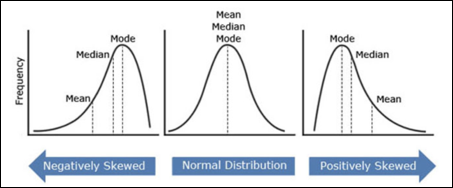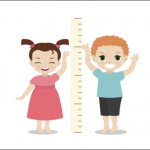The Normal Distribution: The height of a population is one of the famous examples of normal (bell-curve) distribution. Most of the people in a certain population are of average height, and only a very few people are either extremely tall or extremely short. In case of a normal distribution, ideally the mean = median = mode.The Positively Skewed Distribution: This distribution is also called the right skewed distribution. If we study the income of a specific population/region, most of the population’s income would be on the lower side (the left) and a few rich people, who would have extremely high incomes, the tail extending towards the right side. The extreme values are on the right side and the mean shifts towards them. The mean will be greater in value than the median and both the mean, median will be greater in value than the mode.The Negatively Skewed Distribution: This distribution is also called the left skewed distribution. If we study the retirement age, most of the population’s age would be mid-sixties or more. Most people are on the higher side (the right) and only a few people retire at a young age, the tail extending towards the left/lower side. The extreme values are on the left side and the mean shifts towards them. The mean will be lesser in value than the median and both the mean, median will be lesser in value than the mode.

## Let us understand the trick:Let us say that in a neighbourhood, the mean salary of a family is \$ 25,000 (with the effects of extreme values) and we got this by adding up all the income values and dividing it by the number of values. The median salary of the neighbourhood is \$8,000, which means that half of the families in the neighbourhood have salaries less than \$8,000 and half of them have salaries greater than \$8,000. The modal salary is \$10,000, which means that there are more families in the neighbourhood with this income, compared to others.

So, if someone wants to project this neighbourhood as a wealthy one for some reason, he could pick the mean and make a statement like “ The average salary of families in XXX neighbourhood is \$25000”. If someone else wants to get some waiver or lower the price of some service in the neighbourhood, he could pick the median, the lowest among the three and say that “ The average salary of families in XXX neighbourhood is \$8000”. Watch out, the “average” word is the one that is tricky here.

Picture Source : Google

Inspired by the book : ‘How to lie with Statistics’ by Darrell Huff.Priyasha Prasad I am currently doing my Masters in Business Analytics. My topics of interests include Statistics, Econometrics, Database Management, Data Analytics, Machine Learning, Data Visualization, Artificial Intelligence, Data Governance and Ethics, Digital Transformation. I am an Information Technology Professional with 3 years of Technology Consulting experience, as a Business technology Analyst, working in projects from Healthcare, Insurance and Banking domains at Deloitte Consulting. I hold a Bachelor’s degree in Electrical and Electronics Engineering. I enjoy writing, reading books on a variety of topics and blogs on fashion, health and fitness . I am passionate about art & crafts - enjoy making DIY projects, practising doodle art and sewing basic patterns.

4 min read 162